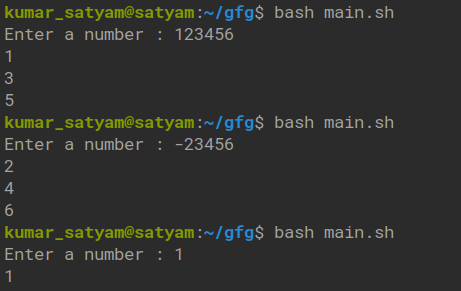# Shell Script to Display the Digits which are in Odd Position

• Difficulty Level : Medium
• Last Updated : 09 Feb, 2022

Here given a list of numbers, our task is to find the odd position from the given number.

Example:

```Input: 123456
Output: 135
Explanation: 1, 2, 3 are at odd position.

Input: 34567
Output: 357
Explanation: 3, 5, 7 are at odd position.```

We will have to input a number from the user for a dynamic script.  After the number input, we need to whether the input is a number or not. We may also accept numbers with signs as well.  We’ll have to input from the user until he/she inputs a pure number. Then we may exactly how many digits are there in the variable, we then need to loop through only the odd positioned digits and print the same. Usage of cut, read, and other basic Linux utility tools to make the task simpler and efficient.

Approach:

• Take input from users.
• Count of the digits.
• Select the particular digits.
• Handle + and – symbols (sign).
• Get the result.

Below is the implementation:

```#!/bin/bash
n=0
while ! [[ \$n =~ "^[-+]?[0-9]+\$" ]]
do
read -p "Enter a number : " n
l=\${#n}
if [[ \$n =~ ^[+-]?[0-9]+\$ ]];then
if [[ \$n =~ ^[+-] ]];then
i=2
if
if [[ \$n =~ ^[0-9] ]];then
i=1
if
while [ \$i -le \$l ]
do
d=\$(echo \$n | cut -c \$i)
echo \$d
i=\$((\$i + 2))
done
break
if
done```

Output:My Personal Notes arrow_drop_up